# Units Master

100+Everyone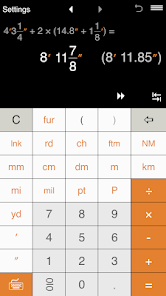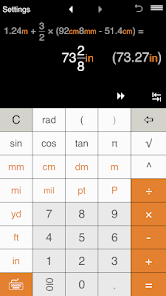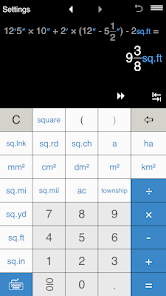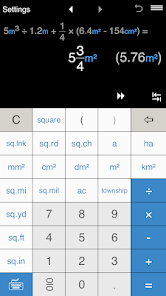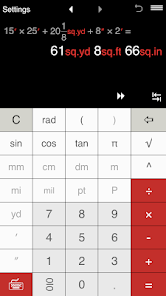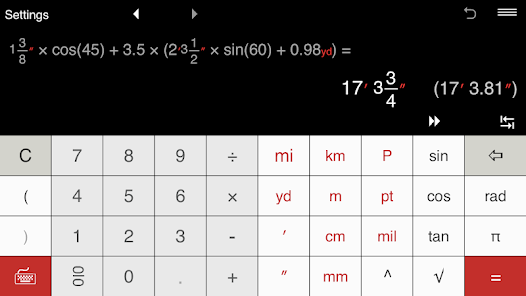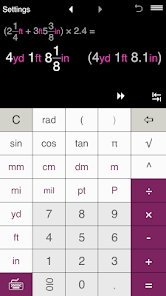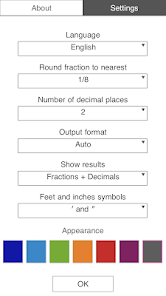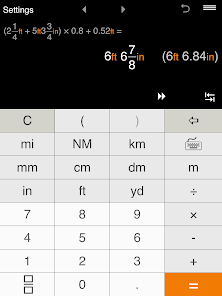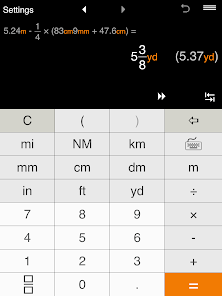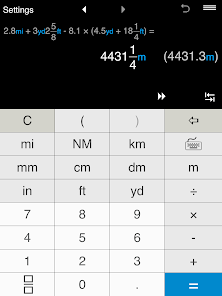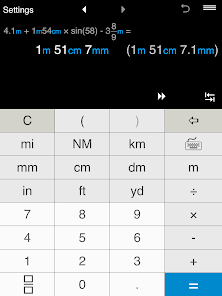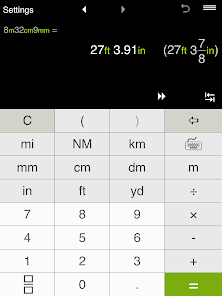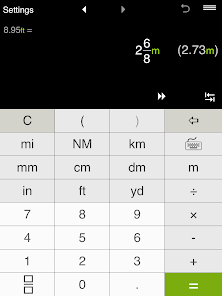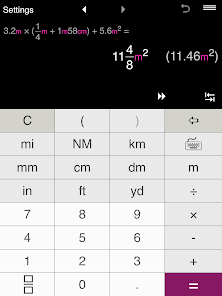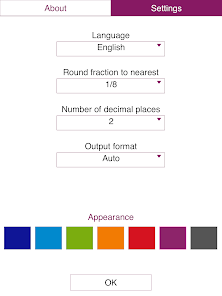Innovative unit conversion calculator.
Get instant help with unit calculation and conversion!
UNITS MASTER is a universal unit conversion calculator that allows you to instantly calculate and convert US and metric units of length, distance and area.

SUPPORTED DISTANCE AND LENGTH UNITS:
US/imperial units: mils, points, picas, inches, links, feet, yards, rods, chains, furlongs, miles, fathoms, nautical miles.
Metric units: millimeters, centimeters, decimeters, meters, kilometers.

SUPPORTED AREA UNITS:
US/imperial units: square mils, square inches, square links, square feet, square yards, squares, square rods, square chains, acres, square miles, townships.
Metric units: square millimeters(mm²), square centimeters(cm²), square decimeters(dm²), square meters(m²), ares, hectares, square kilometers(km²).

LENGTH AND AREA UNIT CALCULATOR:
- Calculates any combination of supported US and metric length and area units.
- Performs calculation in fractions and decimals.
- Complex calculations.
- Easy fraction input.
- Instantly converts results between different units.
- Displays results in fractional and decimal forms.
- Shows answer in any supported unit(s).

LENGTH AND AREA UNIT CONVERTER:
- 1000+ conversion combinations.
- Converts units between US and metric systems.
- Converts between units within the same system.
- Converts between fractions and decimals.

ROUND RESULTS TO SPECIFIED PRECISION:
• Decimal results can be rounded to 0-9 decimal places. E.g. you may round results to the nearest 10ths, 100ths, etc. By default, the app is set up to round to 2 decimal places. If you need to round results to the nearest whole number, select 0 decimal places.
• Rounding fractions to the nearest 1/2, 1/4, 1/8, 1/12, 1/16, 1/32, 1/64, 1/128, 1/256. If you do not need to round fractions, just select "-".

It addition to extensive unit conversion and calculation options, UNITS MASTER offers standard features such as basic operations, calculation with parentheses, calculations with fractions and decimals, exponents, square root, trigonometric functions, etc.

Other features:
• Three virtual keyboards.
• 3 output format for results: Automatic, Metric, US.
• 'Show results' option: Fractions + Decimals, Auto, Fractions, Decimals.
• Choose the way the app shows foot and inch symbols - you may use abbreviations ft and in or ′ for foot and ″ for inch.
• Calculation history to view, recall, edit and share calculations.
• Share calculation results by email.
• 'Undo' for the Clear command.
• Portrait and landscape mode.
• Multiple themes to choose from.

If you have any questions or need help with UNITS MASTER, please visit the online help and FAQ pages:
https://unitsmaster.com/en/help/
https://unitsmaster.com/en/faq.html
Updated on
Aug 13, 2020

## Data safety